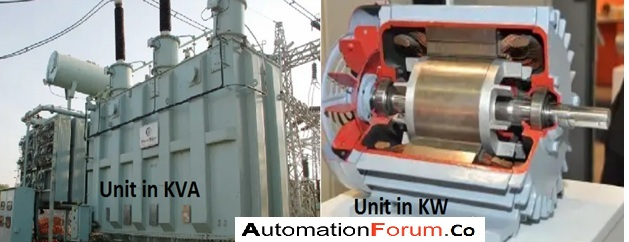# What is the difference between KW and KVA and why the transformer is in kVA?#### What is a watt and what is a kilowatt?

The Si unit of the electric power is watt and 1 watt is the power that is used by an appliance, and the power is consumed when 1A of current flows at a potential difference of 1volt so we can calculate the watt

1 watt = 1 volt × 1 ampere

1000watts = 1 kilowatt

Kilowatt

The electric power that is distributed from the generating station to the consumer is in the kilowatt-hour unit. So 1kwh would be the amount of energy utilized by the appliance if it is operated for 1 hour.

So if a consumer uses two electrical appliances that have 4Kw and 2kw for two hours then the total power utilized is 4+2=6Kw

6×2= 12KW

#### What is the difference between kW and KVA?

The major difference between the kilowatt and kilowatt ampere is the power factor, the power factor can be seen in kW but it is not in kVA. The kVA is the apparent power and the kilowatt is the actual power. So we can consider the KW as the actual power the power that we use and KVA is the power that we get. So this can be explained by using an example we can take the case of the transformer, the transformer is a power source so it is in kVA because it doesn’t have a load and it is the source of power so there is no power factor in it. While in case of the motor we use the unit KW because it has power factor and it is a load it uses the power and how much power can the motor utilize from its source, all of this can be explained and that’s why the motor is in KW and the transformer is in KVA.

KW =VIcosphi

KVA= VI

There is no power factor in the transformer because it is a power source and it is not like a load, so it won’t utilize power. A transformer would have copper and iron loss, the copper loss is because of the transformer winding and this is dependent on the winding resistance and current, iron loss is because of the transformer’s core and this is a constant loss and it is dependent on voltage. So the copper loss is dependent on the current and the iron loss is dependent on the voltage and therefore no power factor.

In the motor, we can see the power factor, consider a motor with 3KW and this will give the 3KW output but in the case of a transformer it is different. So from a 10KVA transformer, we won’t be able to get 10KVA output because there would be many losses. So a transformer or a generator would have a KVA unit because it is a source and they don’t have any direct connection with the load and that’s why they don’t have a power factor. So this is the difference between KW and KVA.

Scroll to Top